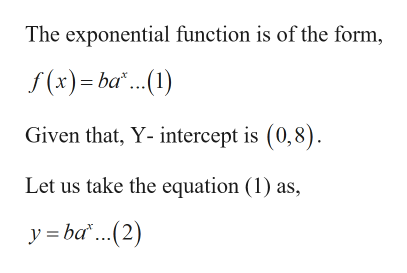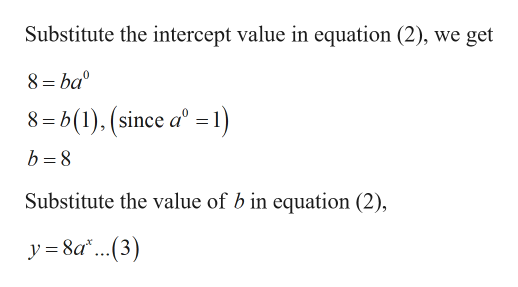# Find an exponential function of the form f(x) = bax that has the given y-intercept and passes through the point P.y-intercept 8;    P(3, 1)

Question
4 views

Find an exponential function of the form f(x) = bax that has the given y-intercept and passes through the point P.

y-intercept 8;    P(3, 1)
check_circle

Step 1

Given:

Step 2

Calculation:

To find the exponential function:help_outlineImage TranscriptioncloseThe exponential function is of the form, f(x) = ba.(1) Given that, Y- intercept is (0,8). Let us take the equation (1) as, y = ba"...(2) fullscreen
Step 3help_outlineImage TranscriptioncloseSubstitute the intercept value in equation (2), we get 8 = ba 8= b(1). (since aº =1) b = 8 Substitute the value of b in equation (2), y = 8a*..(3) fullscreen

### Want to see the full answer?

See Solution

#### Want to see this answer and more?

Solutions are written by subject experts who are available 24/7. Questions are typically answered within 1 hour.*

See Solution
*Response times may vary by subject and question.
Tagged in

### Algebra